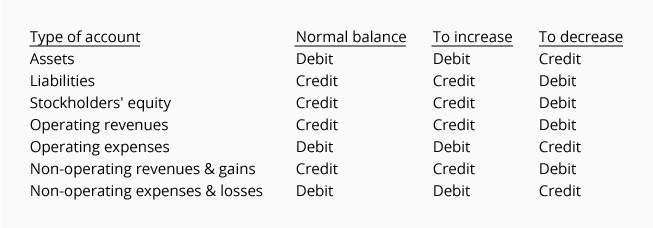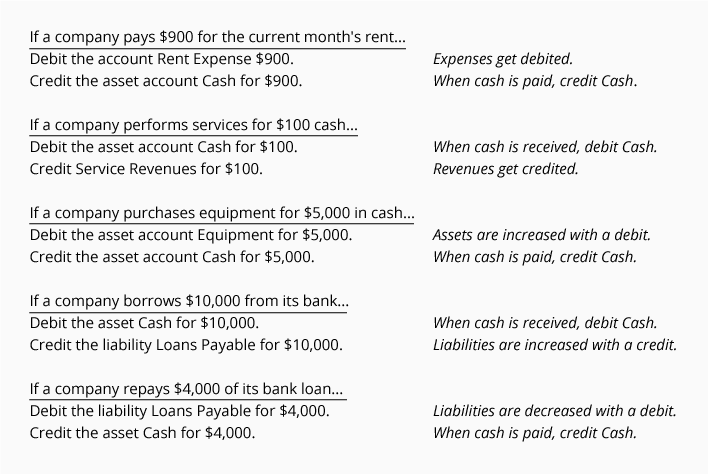# Debits and Credits in the Accounts

If you already understand debits and credits, the following table summarizes how debits and credits are used in the accounts.If you are not familiar with debits and credits or if you want a better understanding, we will provide a few insights to help you. We will also provide links to our visual tutorial, quiz, puzzles, etc. that will further assist you.

### Accounting Equation Can Help

The accounting equation is a central part of bookkeeping and accounting. It can also provide insights into debits and credits. The basic accounting equation is:

Assets = Liabilities + Stockholders' equity (if a corporation)

or

Assets = Liabilities + Owner's equity (if a sole proprietorship)

With double-entry accounting, the accounting equation should always be in balance. In other words, not only will debits be equal to credits, but the amount of assets will be equal to the amount of liabilities plus the amount of owner's equity.

The accounting equation is also the framework of the balance sheet, one of the main financial statements. Hence the balance sheet must also be in balance.

We will use the accounting equation to explain why we sometimes debit an account and at other times we credit an account.

Assets are on the left side of the accounting equation.
Asset account balances should be on the left side of the accounts.

In the accounting equation you can see that assets are on the left side of the equation:Earlier you learned that debit means left side. Recall our T-account that showed debits on the left side:Hence, asset accounts such as Cash, Accounts Receivable, Inventory, and Equipment should have debit balances.

Liabilities are on the right side of the accounting equation.
Liability account balances should be on the right side of the accounts.

In the accounting equation you can see that liabilities are on the right side of the equation:Earlier you learned that credit means right side. Recall our T-account that showed credits on the right side:Thus liability accounts such as Accounts Payable, Notes Payable, Wages Payable, and Interest Payable should have credit balances.

Stockholders' equity is on the right side of the accounting equation.
Stockholders' equity account balances should be on the right side of the accounts.

In the accounting equation you can see that stockholders' equity is on the right side of the equation:Again, credit means right side and our T-account showed credits on the right side. This means that stockholders' equity accounts such as Common Stock, Retained Earnings, and M J Smith, Capital should have credit balances.

Example

To demonstrate the debits and credits of double-entry with a transaction, let's assume that a new corporation is formed and the stockholders invest \$100,000 in exchange for shares of common stock. There are two effects of this transaction:

1. The corporation receives cash, which is recorded as a corporation asset.
2. The corporation issues shares of common stock. The amount received for the shares will be recorded as part of the corporation's stockholders' equity.

Here's how the transaction will impact the accounting equation and the company's balance sheet:Here is what will occur in the general ledger accounts:If this transaction is entered in a general journal, it would appear as follows:Revenues increase stockholders' equity (which is on the right side of the accounting equation).
Therefore the balances in the revenue accounts will be on the right side.

To illustrate, let's assume that a company provides a service and bills the customer \$400 with the amount due in 30 days. Two things occur:

1. Revenues of \$400 are earned and that causes stockholders' equity to increase.
2. The company earns the right to receive \$400. This increases the company's asset account Accounts Receivable.

Here's the effect on the accounting equation and the company's balance sheet as a result of earning the revenues:Here is what occurs in the general ledger accounts:Note: Even though stockholders' equity will increase, the transaction is recorded in the account Service Revenues. The reason is that we want the amount of revenues to be reported on the current period's income statement. (In other words, we temporarily credit Service Revenues instead of crediting the stockholders' equity account Retained Earnings. At the end of the accounting year, the balances in all of the income statement accounts will be closed/transferred to Retained Earnings.)

If this transaction were entered in a general journal, it would appear as follows:Expenses decrease stockholders' equity (which is on the right side of the accounting equation).
Therefore expense accounts will have their balances on the left side.

To reduce the normal credit balance in stockholders' equity accounts, a debit will be needed. Hence, the accounts such as Rent Expense, Advertising Expense, etc. will have their balances on the left side.

For example, when a company pays cash of \$150 for advertising materials that are distributed immediately at a local event, two things occur:

1. An expense of \$150 occurred and the expense will cause stockholders' equity to decrease.
2. The company has reduced its asset Cash by \$150.

The effect on the accounting equation and the company's balance sheet is:The effect on the company's general ledger accounts is shown here:Note: Even though this expense causes stockholders' equity to decrease, the transaction is recorded in the account Advertising Expense. The reason is that we want the current period's income statement to report this expense. (In other words, we temporarily debit Advertising Expense instead of debiting the stockholders' equity account Retained Earnings. At the end of the accounting year, all of the balances in the income statement accounts will be closed/transferred to Retained Earnings.)

If this transaction were entered in a general journal, it would appear as follows:A few tips about debits and credits:

• When cash is received, debit Cash.
• When cash is paid out, credit Cash.
• When revenues are earned, credit a revenue account.
• When expenses are incurred, debit an expense account.

Here are some common transactions with the appropriate debits and credits: